# Unplanned order book details

Unplanned order book data displays on the Revenue forecast chart. This data is drawn from the following sources:

Unplanned order book data is calculated for both fixed-price deliverables and time & materials deliverables, and is based on the time frame indicated by the deliverable’s start and finish dates, excluding any days in the past.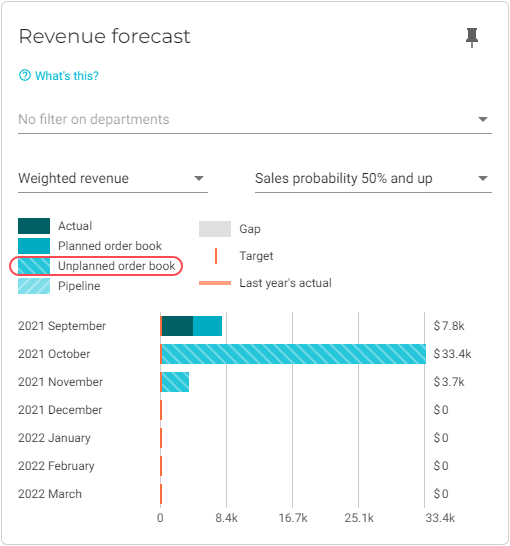To view unplanned order book data for a particular month, click on the month in question.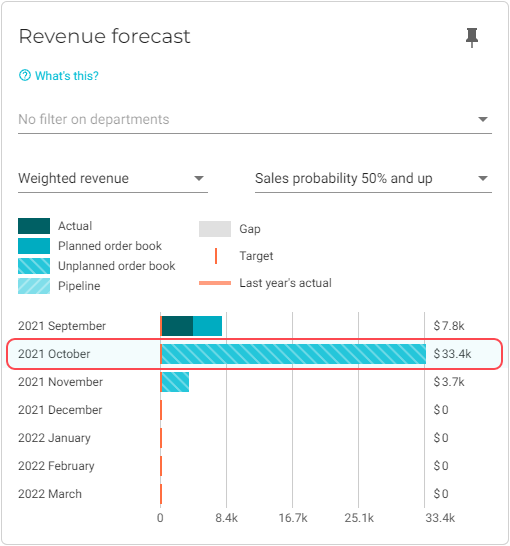The Forecast details screen will display. The total unplanned order book value for the selected month and year to date display on this screen.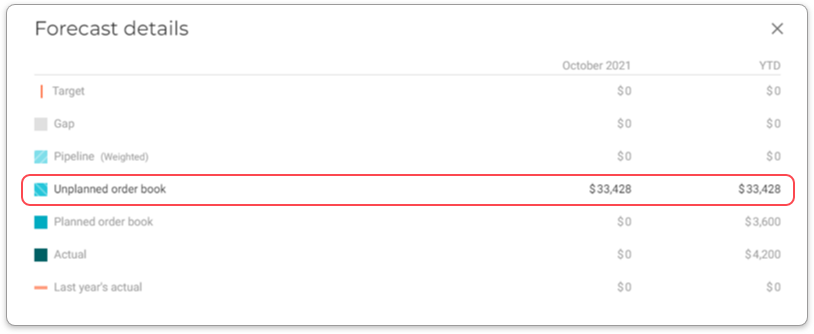To view more details about the unplanned order book forecast, click Unplanned order book. The row will expand to display a list of projects with unplanned deliverables.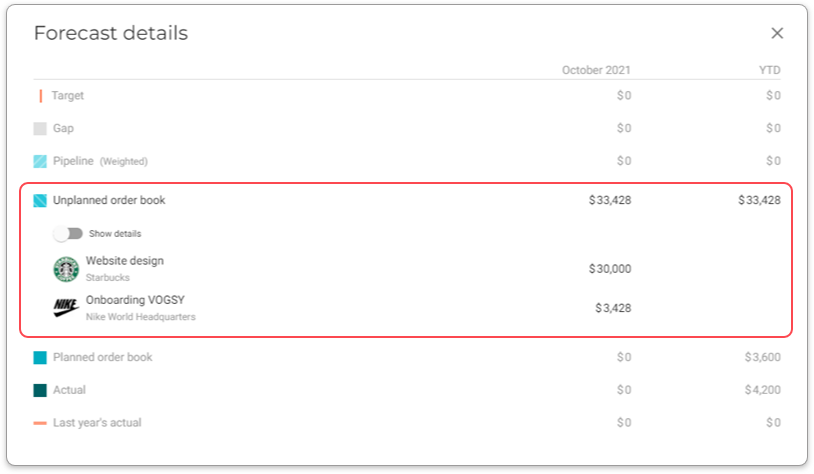To view the project, click on its row.

To view details about the data sources, switch on the Show details toggle.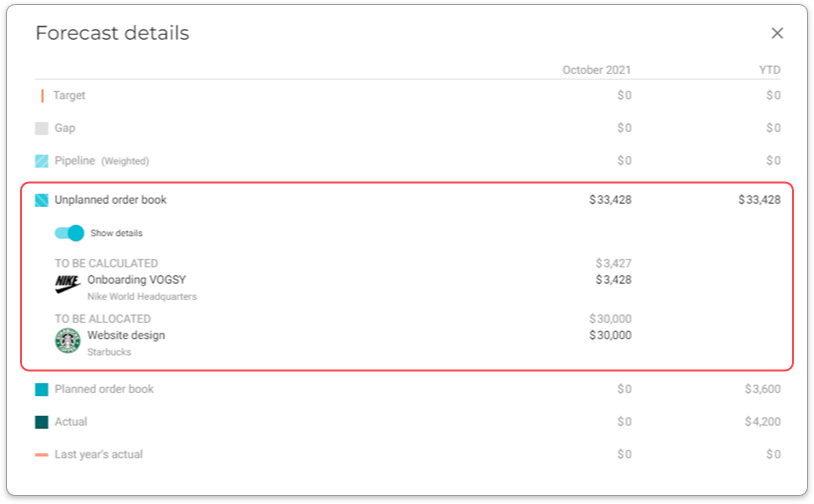The following sections give more details about the Unplanned order book data sources:

## To be calculated

 Applies to fixed price deliverables only.

The value displayed here is the amount to be spent for the selected month.

VOGSY does the following to calculate this value:

1. VOGSY calculates the total calculated sales amount (TCSA), which is the sum of the calculated sales amounts of the deliverable’s activities.

2. VOGSY calculates the delta (D) by subtracting the TCSA from the deliverable’s fixed price amount.

3. VOGSY calculates the numbers of days (N) between the deliverable’s start date and its finish date.

4. VOGSY calculates the deliverable’s daily to be calculated rate (d2BCR) by dividing the D by N.

5. VOGSY calculates the to be calculated amount for the current month by multiplying the d2BCR by the number of days remaining in the current month.

## To be allocated

The value displayed here is based on the current month’s total calculated sales amount minus the value of the current month’s allocated hours.

## To be planned

The value displayed here is calculated in the following manner:

1. For each activity with hours that need to be planned, VOGSY multiplies the to be planned hours by the activity’s sales rate.

2. VOGSY adds the results of the previous step to calculate the total value of the hours to be planned.